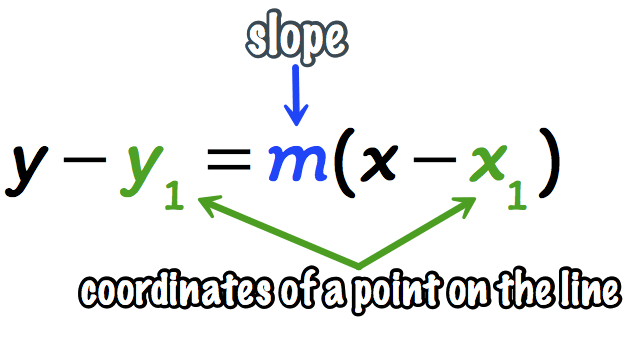# What is the slope and intercept of y-(-4)=-1(x-6)?

Aug 3, 2018

Slope: -1

x-intercept: (2, 0)

y-intercept: (0, 2)

#### Explanation:

$y - \left(- 4\right) = - 1 \left(x - 6\right)$

We know that the equation is in point-slope form:Therefore, we know that the slope is $- 1$.

To find the $x$-intercept, plug in $0$ for $y$ and solve for $x$:
$0 - \left(- 4\right) = - 1 \left(x - 6\right)$

$4 = - x + 6$

$- 2 = - x$

$x = 2$

The $x$-intercept is at $\left(2 , 0\right)$.

To find the $y$-intercept, plug in $0$ for $x$ and solve for $y$:
$y - \left(- 4\right) = - 1 \left(0 - 6\right)$

$y + 4 = - 1 \left(- 6\right)$

$y + 4 = 6$

$y = 2$

The $y$-intercept is at $\left(0 , 2\right)$.

Hope this helps!

Aug 4, 2018

Slope $- 1$, $x$-int $2$ and $y$-int $2$

#### Explanation:

Our equation is in point-slope form

$y - {y}_{1} = m \left(x - {x}_{1}\right)$, where slope $m$ and points (${x}_{1} , {y}_{1}$).

We immediately see that our slope is $- 1$. We can find the $y$-intercept by converting this to slope intercept form

$y = m x + b$, with slope $m$ and a $y$-intercept of $b$.

Our equation simplifies to

$y + 4 = - 1 \left(x - 6\right)$

We can distribute the negative on the right to get

$y + 4 = - x + 6$

Lastly, we can subtract $4$ from both sides to get

$y = - x + 2$

We see that our $y$-intercept is $2$. What about the $x$-intercept? This can easily be found by setting $y$ equal to zero.

$- x + 2 = 0 \implies - x = - 2 \implies x = 2$

Therefore, our slope is $- 1$, our $y$-intercept is $2$, and so is our $x$-intercept.

Hope this helps!